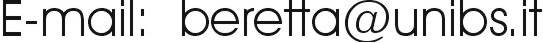Quantum Thermodynamics

2.997- Advanced Topics in Mechanical Engineering

# Brief Course Outline

Explores the foundations of thermodynamics from a quantum theory point of view, by developing a unified theory of mechanics and thermodynamics and a general quantum equation of motion for reversible and irreversible processes from which the second law emerges as a general dynamical theorem.  Topics include non-equilibrium and stable equilibrium states of qubits, qudits, and fermion and boson fields; entanglement, entropy, single-particle quantum heat engines, and open conceptual issues in quantum statistical mechanics and quantum theory of open systems. Emphasis on conceptual definitions. logical consistency, and rigorous mathematical-physics approach.

Self-contained reviews of the necessary background in classical and quantum mechanics, general thermodynamics principles and linear algebra.

Graduate students from all science and engineering courses welcome.

Contact instructor for permission. Email:### Instructor: Gian Paolo Beretta

Course material: handouts plus few papers among those available at http://www.quantumthermodynamics.org/

# Detailed Course Outline

## 2. (14 hrs) KINEMATICS

Definition of the fundamental concepts of

-        system

-        physical observable or property

-        preparation

-        homogeneous vs heterogeneous preparation

-        state

-        mean value of an observable

-        expectation values for a heterogeneous preparation

and their mathematical representations in

-        Classical Mechanics

-        Classical Statistical Mechanics

-        Quantum Mechanics

-        Quantum Statistical Mechanics

-        Quantum Thermodynamics

-        Quantum Statistical Thermodynamics

Observables and measurements

-        quantum (Heinsenberg and Schroedinger-Robertson) uncertainties

-        coherent states

-        complete set or "quorum" of observables

-        measurement of the state, quantum tomography

-        "pure" and "mixed" states

-        correlation

-        entanglement

-        Bloch sphere representation for a two-level system (qubit)

## 3. (22 hrs) DYNAMICS

Hamiltonian dynamics in

-        Classical Mechanics: Hamilton equations of motion

-        Quantum Mechanics: Schroedinger equation of motion

-        Quantum Thermodynamics: von Neumann equation of motion

-        Energy and the generator of Hamiltonian dynamics

-        stability of the equilibrium states

-        time-energy uncertainty relations

(Quantum Thermo)Dynamics of a single constituent of matter: general equation of motion for reversible and irreversible processes

-        generators of the motion

-        constants of the motion

-        nondissipative states and limit cycles

-        equilibrium states

-        conservation of cardinality of the density operator

-        von Neumann entropy functional

-        rate of entropy increase for a strictly isolated and uncorrelated system, irreversibility

-        unstable equilibrium states

-        stable equilibrium states and their uniqueness (second law)

-        Onsager reciprocity relations as general features of the dissipative part of the dynamics

-        application to a two-level system (quibit)

-        application to a d-level system (qudit)

-        time-energy and time-entropy uncertainty relations

-        Carnot and Otto-like quantum heat-engine cycles for a single spin-1/2 system

-        application to a quantum "field" of indistinguishable particles

-        bosons and fermions

-        Bose-Einstein and Fermi-Dirac distributions at stable equilibrium

-        occupation numbers and spontaneous irreversible internal redistribution

(Quantum Thermo)Dynamics of a composite system

-        reduced density operators

-        separability and locality

-        relaxation and decoherence

-        correlations and entanglement

-        entropy-based and other measures of correlation

-        general equation of motion for a composite system and main features

-        relation with master equations of Kossakowski-Lindblad form

## 4. (4-6 hrs) CURRENT OPEN RESEARCH ISSUES

Discussions of the following or other issues at students' request:

-         thermodynamic adiabatic availability versus Hamiltonian unitary availability ("ergotropy")

-         general indicators of entanglement

-         fluctuations improperly interpreted as second law violations

-         Maxwell demons and quantum ratchets

-         single particle tests of quantum theory

-         Schroedinger-Park "paradox" about the concept of state in Quantum Statistical Mechanics